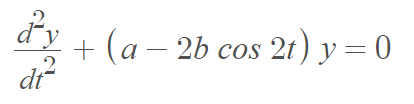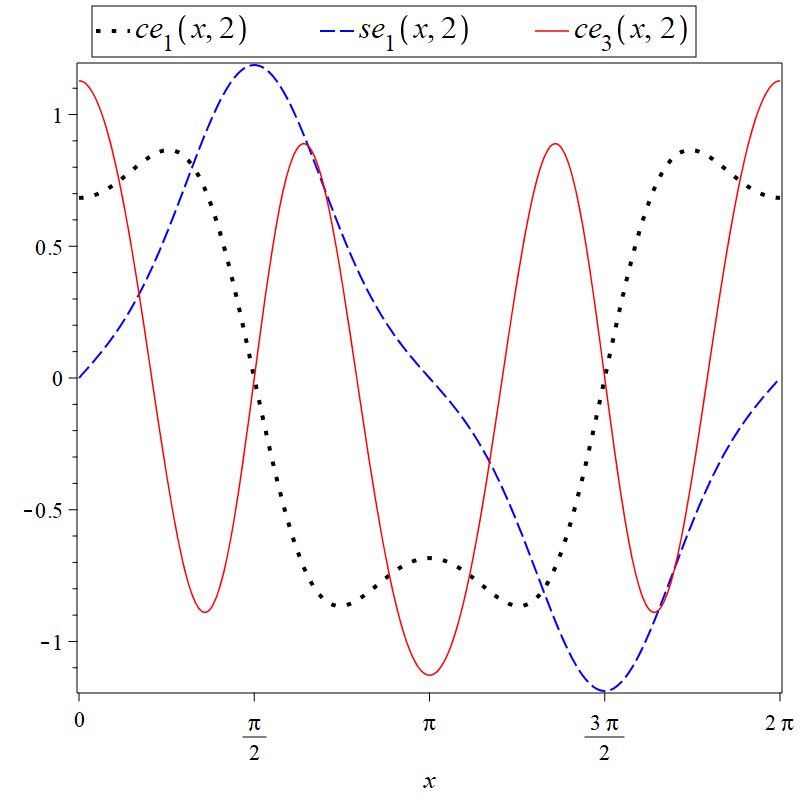# Mathieu Functions: A Gentle Introduction

Mathieu functions (sometimes called angular Mathieu functions) are a series of periodic functions that are solutions to the equation of Mathieu, a second order ordinary differential equation (ODE) defined as 

Where

• η is an elliptic variable (it behaves like a polar angle) with domain 0≤ η < 2π.
• ξbehaves as a radial variable, with domain 0 ≤ ξ < infin;. ξ = 0 corresponds to the line joining the foci (± f, 0)

Mathieu functions are solutions for arbitrary constant values. Solutions may be:

• Even Φm = cem (ηq), m = 0, 1, 2, … or
• Odd Φm = sem (ηq), m = 1, 2, 3 ….

Where m is the order, ce = cosine-elliptic and se = sine-elliptic.

## History and Applications of the Mathieu Functions

The Mathieu equation, an classic equation of non-linear vibrations theory, was introduced by Émile Mathieu (1835-90) during a discussion of an elliptic drum membrane’s vibrations . The equation differs from a simple harmonic oscillator in that it adds a periodic (time-varying) stiffness coefficient a. This coefficient corresponds to the square of its natural frequency .

As well as vibration theory, Mathieu’s equation is used in a wide variety of applied mathematics and engineering, including stability of ships and trains. There are two main categories of physical problems where the equation is used :

• Elliptic geometries: For example, vibrating modes in elliptic membranes, oscillations of water in a lake that is elliptic-shaped, or propagating modes in elliptic pipes. The equations arise when separating the wave equation using elliptic coordinates.
• Periodic motion: an electron’s trajectory in a periodic atom array, oscillations of floating ships, quantum pendulum mechanics.

More complex situations where the function is used include degenerate cases of ellipses (e.g., scattering from conductive strips) or scattering from dielectric elliptic cylinders .

## Challenges with Mathieu Functions

Mathieu functions have relatively rich behavior and can be challenging to understand. To compound the problem, five different naming conventions are in use due to McLachlan & Gradshteyn , Erdelyi , Morse, Abramowitz, and Stratton ; Some conventions may affect the normalization of the function in addition to their labels . You may also see a variety of different constants like a & b like this variant .Perhaps the biggest challenge is that the analytic solutions to the Mathieu function are extremely challenging to find and use, “mainly because of the impossibility of analytically representing them in a simple and handy way” . Built in functions and/or routines are not widespread. When functions do exist, they may be slow to converge or lack any kind of convergence at all . More complicated function behavior is often easier to understand with 2D and 3D plots.## References

 Gutierrez-Vega, J. et al. (2003). Mathieu functions, a visual approach. Retrieved March 7, 2022 from: https://www.academia.edu/4329322/Mathieu_functions_a_visual_approach
 Mathieu, E.L. Journal de Mathematiques, Vol. 13 (2) , 1868, p. 137.
 Kovacic, I. et al. Mathieu’s Equation and Its Generalizations: Overview of Stability Charts and Their Features. Retrieved March 7, 2022 from: http://audiophile.tam.cornell.edu/randpdf/AMR.pdf
 Peterson, A. & Bibby, M. (2013). Accurate Computation of Mathieu Functions. Morgan & Claypool Publishers.
 N. W. McLachlan, Theory and Application of Mathieu Functions. Oxford Press, London, 1951; I. S. Gradshteyn and I. M. Ryzhik, Table of Integral Series and Products,
6th ed. Academic, London, 2000, Chap. 8.
 A. Erdelyi, Bateman Manuscript Project on Higher Transcendental Functions. McGraw-Hill, Malabar, FL, 1981, Chap. 16.
 P. M. Morse and H. Feshbach, Methods of Theoretical Physics.[PDF] McGraw Hill, New York, 1953, Chap. 6.
 M. Abramowitz and I. Stegun, Handbook of Mathematical Functions. [PDF] Dover, New York, 1964, Chap. 20.
 J. A. Stratton, Electromagnetic Theory. McGraw-Hill, New York, 1941, Chap. 6
 Davis, H. (1960). Introduction to Nonlinear Differential and Integral Equations. United States Atomic Energy Commission. PDF.
 Sips, R. “Représentation asymptotique des fonctions de Mathieu et des fonctions d’onde sphéroidales.” Trans. Amer. Math. Soc. 66, 93-134, 1949.

CITE THIS AS:
Stephanie Glen. "Mathieu Functions: A Gentle Introduction" From StatisticsHowTo.com: Elementary Statistics for the rest of us! https://www.statisticshowto.com/mathieu-functions-a-gentle-introduction/